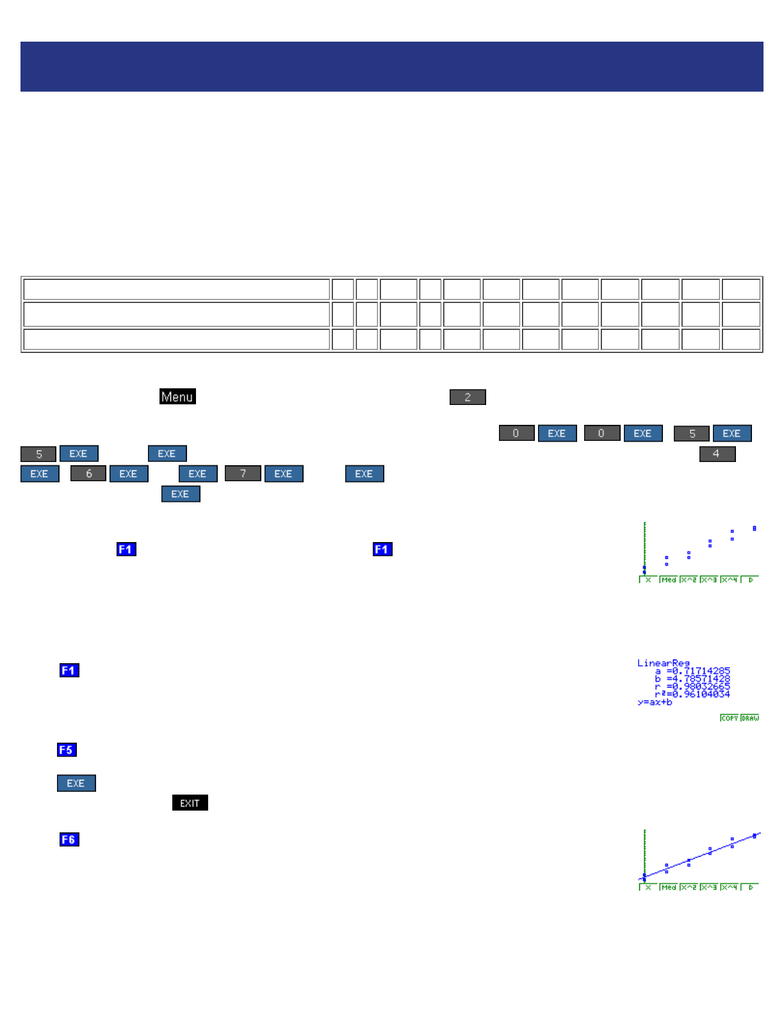Class Notes (1,100,000)
CA (630,000)
Ryerson (30,000)
QMS (200)
QMS 202 (60)
Lecture

QMS 202 Lecture Notes - Saccharomyces Cerevisiae, Linear Regression, Yeast

Department
Quantitative Methods
Course Code
QMS 202
Professor
Charles Barkley

This preview shows half of the first page. to view the full 3 pages of the document.Casio CFX-9850 TechSkills 1: Functions
http://www.prenhall.com/esm/app/calc_v2/calculator/medialib/Technology/Documents/CFX9850/desc_pages/cfx9850techskills2.html[11/6/2012 4:42:02 PM]
Casio CFX-9850
TechSkills 2: Regression
In this TechSkills module, we take a given data set and fit an appropriate function to the data. The formal process of
fitting a function to a given data set is called regression. Regression is a formal component of statistics. We start with
fitting the simplest type of function, a linear function.
Linear Regression
Linear regression is the process of fitting a straight line to a data set. A linear function has the form y = ax + b. Hence,
the task of linear regression is to determine values for a and b that create the straight line that best fits the given data.
For example, we consider a data set from Sullivan and Sullivan, Precalculus enhanced with graphing utilities, Second
Edition, page 112, Example 3.
Plot 1 2 3 4 5 6 7 8 9 10 11 12
Fertilizer, X (lbs/100ft2)0 0 5 5 10 10 15 15 20 20 25 25
Yield, Y (bushels) 4 6 10 7 12 10 15 17 18 21 23 22
Our first task is to enter the data set into the Casio. Fortunately, the Casio makes this a simple task. Turn the calculator
on, and then press the key to display the MAIN MENU. Press to select the STAT menu. A spreadsheet is
now displayed. Enter the fertilizer amounts in List 1 and the corresponding yields in List 2. Use the thumb pad to
navigate from List 1 to List 2. To enter the fertilizer amounts in List 1, press , , ,
, ..., 25 . Then use the thumb pad to navigate to List 2. To enter the yields in List 2, press
, , 10 , ,..., 22 . Note that a specific number is not actually placed into the
list until you press the key.
After the data set has been entered and checked for accuracy, we are ready to see a plot of the
data. Press the key to access the GRPH menu. Press to select GPH1, statistical graph 1. A
plot of the data set appears.
Notice that it looks reasonable to fit a straight line through the displayed data points. A suite of regression types
appears at the bottom of the screen.
Press to select X, the CasioÕs symbol for linear regression. The screen then displays the
LinearReg screen with the values for the linear form y = ax+b for the straight line of best fit.
Here, to three decimal places, the linear equation of best fit is y = .717x + 4.786.
Press to select COPY to copy the linear regression equation into the Graph Func editor.
Press to store the regression equation as function Y1 in the Graph Func menu and return to the LinearReg
screen; otherwise, press to return to the LinearReg screen.
Press to select DRAW. The Casio then displays the linear regression line superimposed on the
data.
Of course there are other functional relationships besides linear. The Casio supports a handy suite of choices, including
linear, quadratic, cubic, quartic, logarithmic, exponential, power, sinusoidal and logistic.
You're Reading a Preview

Unlock to view full version